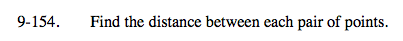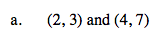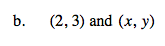Home > A2C > Chapter 9 > Lesson 9.3.2 > Problem9-154

9-154.
1. Find the distance between each pair of points. Homework Help ✎

1. (2, 3) and (4, 7)

2. (2, 3) and (x, y)Use the distance formula to find the distance.

$\textit{d}=\sqrt{(4-2)^{2}+(7-3)^{2}}$

$\textit{d}=2\sqrt{5}$See part (a).